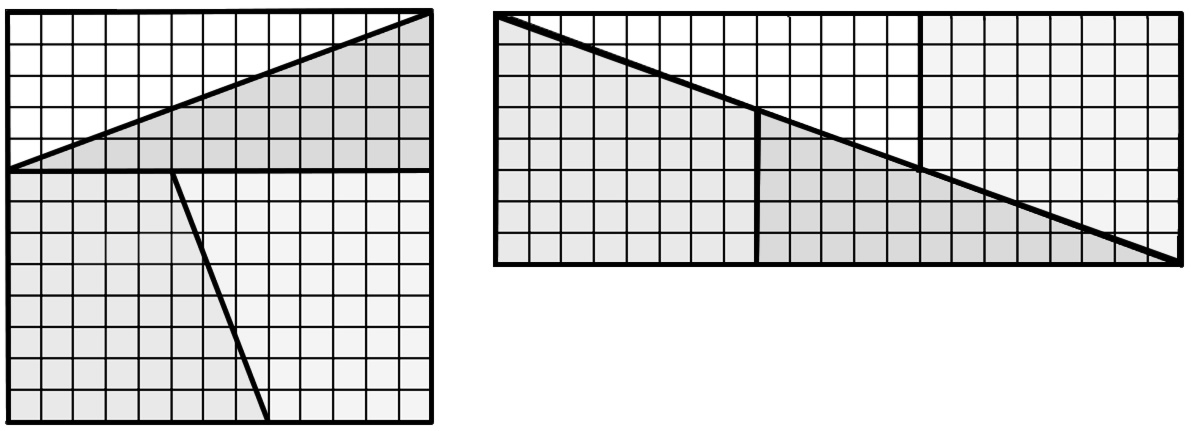# 337

This number is a prime.The smallest prime formed from the concatenated factors of a repunit (3 * 37).337 = 2^(2^3) + 3^(2^2). [Kulsha]813 + 1623 = 97. Note the exponents. [Rubin]Starting with prime 337, the alphabet code value of EMIRP is emirp 1741. [Poo Sung]The first occurrence of two consecutive 3-digit emirps in the sequence of primes. [Beedassy]337# - 1 is a primorial prime. [Patterson]The canvas of the famous painting 'The Night Watch' by Rembrandt van Rijn, weighs 337 kilograms. [Bakker]The sum of the areas of the rectangles in this Fibonacci paradox: if we divide a 13-by-13 square into four pieces as shown below, they can apparantly be rearranged into the one unit smaller 8-by-21 rectangle.[Caldwell]3!3! -/+ 7 form consecutive primes. [Beedassy]The smallest 3-digit prime that is an absolute, prime-digit, left-truncatable prime (and of course, an emirp and circular prime). [Green]The smallest emirp which when prefixed to the smallest pandigital string forms another emirp: 3370123456789. [Beedassy]The mean of the first 337 square numbers is itself a square. This is a first occurrence. [Jones]"Show that J_3 = 3 Pi Zeta(3) and hence that there are, on average, about 337 neutrinos, left over from the Big Bang, in each cubic centimetre of your Universe." Reference: David Broadhurst, Feynman's sunshine numbers. [Post]Constantine I died in the year 337, after ruling for 31 years. He was the first of 11 Emperors named Constantine to rule the Byzantines. Note that 11, 31, 337, and their reversals are all primes!A penguin known as No. 337 scaled a rock wall and escaped from the Tokyo Aquarium. [Reuters](337, 347) is the only pair of 3-digit successive primes, (emirps), ending with the same digit. [Loungrides](337, 373) is the only known case of a pair of primes formed by concatenating in order and reverse order the prime factors of a repunit number, R3=111, (111=3*37=37*3). [Loungrides]337 is a permutable prime. 337, 373, 733 are prime. [Sonye]The smallest prime (the only emirp) of form a^b+c^d, where the bases are prime and the exponents are composite digits, i.e., 2^8+3^4. [Loungrides]The only emirp of form a^b + c^d, where the bases are distinct prime digits and the exponents are distinct composite digits, i.e., 2^8+3^4. Alternating the exponents, i.e., 2^4+3^8 we take the prime 6577. [Loungrides]InterContinental Shanghai Wonderland has 337 rooms and suites. [USA Today]Number of sphenic twins up to ten thousand. [Renner]

(There are 14 curios for this number that have not yet been approved by an editor.)